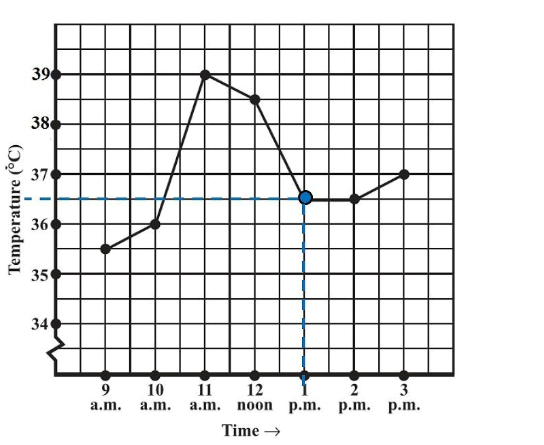# Ex.15.1 Q1 Introduction to Graphs Solutions - NCERT Maths Class 8

Go back to  'Ex.15.1'

## Question

The following graph shows the temperature of a patient in a hospital, recorded every hour.

(a) What was the patient’s temperature at $$1\;\rm{pm}$$?

(b) When was the patient’s temperature $$38.5^\circ\rm{C}$$?

(c) The patient’s temperature was the same two times during the period given. What were the two times?

(d) What was the temperature at $$1.30\;\rm{pm}$$? How did you arrive at your answer?

(e) During which periods did the patient’s temperature show an upward trend?

Video Solution
Introduction To Graphs
Ex 15.1 | Question 1

## Text SolutionReasoning:

Horizontal axis represents Time and vertical represents Temperature in $$^\circ\,\rm{C.}$$

We can find the value of ‘Temperature’ on vertical axis according to the given value of ‘Time’ on the horizontal axis value or vice versa.

Steps:

(a) At $$1\;\rm{ pm}$$ the patient’s temperature was $$36.5^\circ\,\rm{ C.}$$

(b) The patient’s temperature was $$38.5^\circ\,\rm{ C}$$ at $$12$$ noon.

(c) The patient’s temperature was $$36.5^\circ\rm{C}$$  at $$1 \,\rm{pm}$$ and $$2 \,\rm{pm.}$$

(d) The graph between the times $$1 \,\rm{pm}$$ and $$2 \,\rm{pm}$$ is parallel to the x-axis. The temperature at $$1 \,\rm{pm}$$ and $$2 \,\rm{pm}$$ is $$36.5^\circ \;\rm{C.}$$ So, the temperature at $$1.30 \;\rm{pm}$$ is $$36.5^\circ \;\rm{C.}$$

(e) During $$9$$am to $$10 \;\rm{pm}$$, $$10 \;\rm{pm}$$ to $$11 \;\rm{pm}$$, $$2\;\rm{pm}$$  to $$3\;\rm{pm}$$ , the patient’s temperature showed an upward trend.

Learn from the best math teachers and top your exams

• Live one on one classroom and doubt clearing
• Practice worksheets in and after class for conceptual clarity
• Personalized curriculum to keep up with school